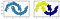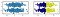# Clustering: A Deeper Look

Clustering is an unsupervised learning technique in which we are trying to find groupings in the data set based on some known or unknown properties which might exist. Irrespective of which technique you may use these are the things we are trying to achieve:

• Within a cluster the points should be similar to each other.
• Between two clusters you need to have a dissimilarity.

Depending on how we define these similarities and dissimilarities there are a number of techniques which one can use. Let us make the problem more concrete.

Where d is the Eucledian distance between two points. We want to find partitions which minimize L. Let us think about the problem in more detail. Suppose you want to divide 4 points into 2 clusters. How many such partitions can we have? For each point we have two choices and we are not considering all points in one cluster and no point in a cluster. So that becomes 2²/2. We can increase the number of points to n and number of clusters to K so that the total partitions become [K^(n-K)]/K!

Now we get close to understanding the complexity of this problem. We can get some estimates by doing an exhaustive search but it becomes very difficult to find the optimal solution.

All solutions in this case are actually sub optimal solutions.

If we think about only two clusters for the time being and we have already found their centers denoted by y₁ and y₂. Now suppose if we want to associate another point with one of the clusters, we have to find the distance between the point and centers of the clusters. We assign a point to a cluster whose distance from center is minimum from the point.

In the diagram above we want to assign the red point to one of the clusters. We can calculate its distance from y1 and y2 and assign it accordingly. What we can also do is we draw a perpendicular bisector of the straight line joining y1 and y2. Then all the points lying on one side of ther perpendicular bisector belong to one of the clusters. Basically, we are creating sets called half spaces. These half spaces are convex. There is an analytical proof for this but if you consider any two points in a half space and join them by a line segment, all points on the line segment will be completely contained within that half space. Now, if we have more than two clusters, we will have more half spaces. And intersection of these half spaces will be again convex. So, this method provides convex shaped clusters. It should be noted that we are still not talking about shape of the finitely many points in the cluster.

There’s also a more subtle point here. Why did we use L₂ norm instead of L₁ norm for calculting distance between the two points in the loss function? As seen in the previous example in case of a 2 dimensional space, the half space is obtained easily by the perpendicular bisector of the line joining the cluster centres. A line has zero measure in the Lebesgue sense. In the case of L₁ norm there will be many points which have the same distance from both cluster centres and such a surface has infinite Lebesgue measure.

Let’s look at some examples and see that just by closely looking at the properties of the loss function we can predict so many useful things.

`from sklearn.datasets import make_moonsfrom sklearn.cluster import KMeansX, y = make_moons(500, noise=.1, random_state=0)labels = KMeans(2, random_state=0).fit_predict(X)fig, ax = plt.subplots(1, 2, sharey=True)ax.scatter(X[:, 0], X[:, 1],edgecolor='k')ax.scatter(X[:, 0], X[:, 1], c=labels,            s=50, cmap='plasma', edgecolors='k')`Fig : Kmeans clustering performance on non convex shapes

If we look at the figure on the left, without even writing a single line of code we can predict that KMeans clustering will not be able to give us the clusters we want since it can only generate convex clusters. The convex hull of theses shapes has a finite region of intersection and thus it will not work.

`import numpy as npcov = [[20,0],[0,1]]x_1, y_1 = np.random.multivariate_normal((1,2), cov, 500).Tx_2, y_2 = np.random.multivariate_normal((1,-3), cov, 500).TX_1 = np.array([x_1,y_1]).TX_2 = np.array([x_2,y_2]).TX = np.vstack((X_1,X_2))labels = KMeans(2, random_state=0).fit_predict(X)fig, ax = plt.subplots(1, 2, sharey=True)ax.scatter(X[:, 0], X[:, 1], edgecolors='k')ax.scatter(X[:, 0], X[:, 1], c=labels, cmap='plasma', edgecolors='k')`Fig : Gaussian with unequal variance in different directions

The centre of one Gaussian is (1,2) and the other is (1, -3). Now ideally these should have been the cluster centres as well. But instead we got something else. If we place the cluster centres at Gaussian centres the sum of distances of different different points from their respective centres would be larger than if the clusters were placed at (-5, 0) and (5,0) like the image on the right. Since the spread in x-direction is greater than the spread in y-direction. So, although these two Gaussian distributions can be enclosed by convex clusters, the clusters we get out of KMeans are still not the one we want.

Another important factor to keep in mind is the density of points in a cluster. Otherwise, the calculation of loss can also be skewed towards higher density regions.

Moral of the story is there should be a match between what you are trying to measure and what the loss function of any technique measures. Otherwise, the output and expectation will always be different.

Data Scientist@ThoughtWorks

## More from Anirudh Gupta

Data Scientist@ThoughtWorks